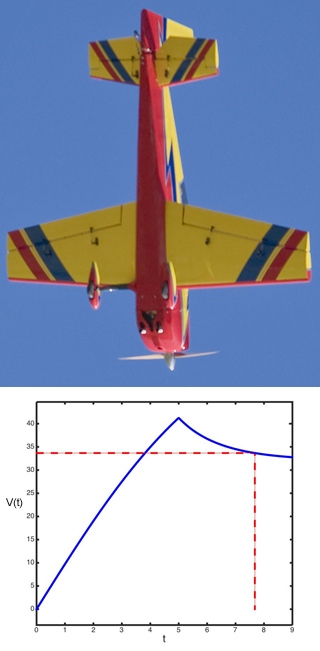2.087 | Fall 2014 | Undergraduate

# Engineering Math: Differential Equations and Linear Algebra

#### Course Description

This course is about the mathematics that is most widely used in the mechanical engineering core subjects: An introduction to linear algebra and ordinary differential equations (ODEs), including general numerical approaches to solving systems of equations.

## Course Info

##### Learning Resource Types
Exams
Lecture Videos
Problem Sets
Programming Assignments with ExamplesA model aircraft is pointed straight down with its engines off. After five seconds, it deploys its speedbrakes. This is a graph of its velocity. See Homework 1 Problem 9 for the full problem and a MATLAB script modeling the solution. (Photograph courtesy of Dean Ritola on flickr.  License: CC BY-NC.)